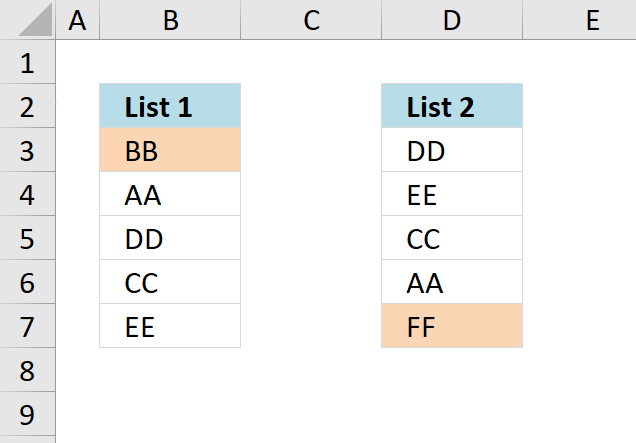Author: Oscar Cronquist Article last updated on November 18, 2018The picture above shows two lists. How do I highlight values in List 1 that are not in List 2? And vice versa? To do this I use conditional formatting.

To extract a list of missing values using excel formula, check this blog post What values are missing in List 1 that exists i List 2?

The conditional formatting formula applied to B3:B7:

=COUNTIF(\$D\$3:\$D\$7, B3)=0

The conditional formatting formula applied to D3:D7:

=COUNTIF(\$B\$3:\$B\$7, D3)=0

Explaining CF formula applied to B3:B7

The COUNTIF function counts the number of time the value in cell B3 exists in cell range D3:D7.

 Cell COUNTIF Result B3 COUNTIF(\$B\$3:\$B\$7, B3) 0 B4 COUNTIF(\$B\$3:\$B\$7, B4) 1 B5 COUNTIF(\$B\$3:\$B\$7, B5) 1

Now if the COUNTIF function returns 0 (zero) that means that the value is not found in the other cell range and the logical expression returns TRUE.

COUNTIF(\$D\$3:\$D\$7, B3)=0

becomes

0=0

and returns TRUE.

Cell B3 is highlighted, the value is not found in the other list.

Get excel example file
lookup-between-two-lists-of-data-to-highlight-missing-data.xls
(Excel 97-2003 Workbook *.xls)